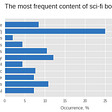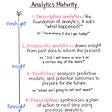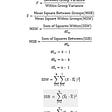# Basic Data Science and Statistic for Beginners

Today’s market is changing in incredible ways with an increased buzz around AI and machine learning. Data science assists these new technologies by figuring out solutions to problems by linking similar data for future use.

# Need Of Data Science:

Traditionally , The Data we had was mostly structured and small in size which could be analysed by using simple BI Tools. But today most of the data is unstructured or semi-structured , so simple BI Tools are not capable of processing this huge volume and variety of data. This is why we need more complex and advance analytical tools and algorithm for processing ,analysing and drawing meaningfull insights out of it.

Let’s have a look at the data trends in the image given below which shows that by 2020, more than 80 % of the data will be unstructured.
Flow of unstructured data

# What is Data Science?

Data science is the field of study that combines domain expertise, programming skills, and knowledge of mathematics and statistics to extract meaningful insights from data. It is a blend of various Tools, Algorithm and Machine learning principle with the goal to discover hidden pattern from raw data.

Data Science is multidisciplinary field which comparises of Maths, IT knowledge and your domain.

Data Science is primarily used to make decisions and predictions making use of predictive causal analytics, prescriptive analytics (predictive plus decision science) and machine learning.

• Predictive causal analytics — If you want a model that can predict the possibilities of a particular event in the future, you need to apply predictive causal analytics. Say, if you are providing money on credit, then the probability of customers making future credit payments on time is a matter of concern for you. Here, you can build a model that can perform predictive analytics on the payment history of the customer to predict if the future payments will be on time or not.
• Prescriptive analytics: If you want a model that has the intelligence of taking its own decisions and the ability to modify it with dynamic parameters, you certainly need prescriptive analytics for it. This relatively new field is all about providing advice. In other terms, it not only predicts but suggests a range of prescribed actions and associated outcomes.
The best example for this is Google’s self-driving car which I had discussed earlier too. The data gathered by vehicles can be used to train self-driving cars. You can run algorithms on this data to bring intelligence to it. This will enable your car to take decisions like when to turn, which path to take, when to slow down or speed up.
• Machine learning for making predictions — If you have transactional data of a finance company and need to build a model to determine the future trend, then machine learning algorithms are the best bet. This falls under the paradigm of supervised learning. It is called supervised because you already have the data based on which you can train your machines. For example, a fraud detection model can be trained using a historical record of fraudulent purchases.
• Machine learning for pattern discovery — If you don’t have the parameters based on which you can make predictions, then you need to find out the hidden patterns within the dataset to be able to make meaningful predictions. This is nothing but the unsupervised model as you don’t have any predefined labels for grouping. The most common algorithm used for pattern discovery is Clustering.
Let’s say you are working in a telephone company and you need to establish a network by putting towers in a region. Then, you can use the clustering technique to find those tower locations which will ensure that all the users receive optimum signal strength.

Let’s see how the proportion of above-described approaches differ for Data Analysis as well as Data Science. As you can see in the image below, Data Analysis includes descriptive analytics and prediction to a certain extent. On the other hand, Data Science is more about Predictive Causal Analytics and Machine Learning.

# What is Data ?

Data are characteristics or information, usually numerical, that are collected through observation. In a more technical sense, data are a set of values of qualitative or quantitative variables about one or more persons or objects.

# What Are Quantitative and Qualitative Data Types in Statistics?

the highest level that gives us 2 kinds of data:

• Quantitative data
• Qualitative data

Quantitative data is information about quantities of things, things that we measure, and so we describe them in terms of numbers. As such, quantitative data are also called Numerical data.

On the other hand, Qualitative data give us information about the qualities of things. They are observed phenomenon, not measured, and so we generally label them with names. Qualitative data are also known as Categorical data.

There are 2 types of Quantitative data — Discrete data and Continuous data

Discrete data is information that can only take certain values and can’t be made more precise. This might only be whole numbers, like the numbers on a die (any number from 1 to 6) or could be other types of fixed number scheme, such as shoe sizes (2, 2.5, 3, 3.5, etc.). They are called discrete data because they have fixed points and measures inbetween do not exist (you can’t get 2.5 on a die, nor can you have a shoe size of 3.49).

Continuous data is data that can take any value, usually within certain limits, and could be divided into finer and finer parts. A person’s height is continuous data as it can be measured in metres and fractions of metres (centimetres, millimetres, nanometres). Time of an event is also continuous data and can be measures in years and divided into smaller fractions, depending on how accurately you wish to record it (months, days, hours, minutes, seconds, etc.)

## Qualitative

o Nominal

o Ordinal

Nominal data : Nominal data is related to identification of categories. Common examples include male/female, hair color, nationalities, and names of people.For example, race is a nominal variable having a number of categories, but there is no specific way to order from highest to lowest and vice versa.

Ordinal Data : Ordinal data is a type of categorical data with an order. The variables in ordinal data are listed in an orderly manner. For example, ranks (1,2,3)

# What is Statistics ?

Statistics is the study of the collection, analysis, interpretation, presentation, and organization of data. In other words, it is a mathematical discipline to collect, summarize data.

# How is statistics used in Data Science ?

Statistical analysis is the science of collecting data and uncovering patterns and trends. It is needed for following tasks:

●Simplifying mass of data : Statistics helps to convert mass of data into significant figures that makes analysis easier.

●For Making future predictions based on past behavior.

● Presenting facts in definite format : Stats enables us to present general statements in a precise and definite format like numbers . Numerical values are more informative than statements.

● Facilitating comparisons of data : Statistics enables comparison between two similar entities by using their individual data and figures.

● Statistical methods help to formulate and test hypothesis to develop new theories.

# Types of Statistics

Basically, there are two types of statistics.

• Descriptive Statistics
• Inferential Statistics

Descriptive Statistics uses the data to provide descriptions of the population, eitherthrough numerical calculations or graphs or tables.

For example , We want to know the average marks of students in a classroom, In descriptive statistics we will record the marks of each and every student in the class and then find out the maximum, minimum and average marks of the class.

Inferential Statistics makes inferences and predictions about a population based on a sample of data taken from the population. Example, Now we want to know the average marks of all the students studying in the same grade like 11th standard . Now it will be difficult to record marks of each student separately . So ,in Inferential Statistics, we will take a sample from the whole population and consider this sample for our statistical study (calculating average marks) for studying the population .

# Understanding Descriptive Analysis

Descriptive statistics helps to describe and understand the features of a specific dataset . With descriptive statistics you are simply describing what is or what the data shows.

## Univariate and Bivariate Analysis

1. Univariate data — This type of data consists of only one variable . The analysis of univariate data is thus the simplest form of analysis since the information deals with only one quantity that changes. It does not deal with causes or relationships and the main purpose of the analysis is to describe the data and find patterns that exist within it. For example, height .There is only one variable that is height and it is not dealing withany cause or relationship.

2. Bivariate data — This type of data involves two different variables . The analysis of this type of data deals with causes and relationships and the analysis is done to find out the relationship among the two variables. Example of bivariate data can be temperature and ice cream sales in summer season.

Descriptive statistics consists of two basic categories of measures: measures of central tendency and measures of variability or spread.

## Measures of central tendency :

● Measures of central tendency provide information about typical or average values of a data set.

● They describe the center of a data set.

Mean, Median and Mode are the most commonly used measures of central tendency.

Mean : It is the average which is simply defined as the ratio of the summation of all values to the number of items. Let’s look at an example of simple set of data representing the age of 10 boys, 4,6,8,12,3,11,7,10,14,9 .The mean weight is calculated as,

Mean = (4+6+8+12+3+11+7+10+14+9) / 10 = 8.4

Mean Formula = ΣX ÷ N

ΣX= Sum of all the individual values,

N= Total number of items

Median : It is essentially known as the central value of a series. Median of a set of values can be arrived only after sorting the data in either ascending or descending order

X = 6, 8,16, 9, 11, 12,5

Sorted X = 5,6,8,9,11,12,16

Median of X = 5,6,8,9,11,12,16

Median = 9

When the count of numbers is even: Median= (n/2) +1

When the count of numbers is odd: Median= (n+1)/2

(n is the count of numbers in the given data)

Mode : Mode is the most frequently occurring number in the dataset. Let us take an example of mode 90, 54, 10, 50, 10, 92, 56.Here in these varied observations the most occurring number is 11, hence Mode=10.

## Measures of dispersion :

Dispersion is used to measure the variability in the data or to see how spread out the data is.In simple words dispersion in statistics is a way of describing how spread out a set of data is.

The spread of a data set can be understood by a range of descriptive statistics including variance, standard deviation, and interquartile range. Spread can also be shown in graphs: dot plots, box plots, and stem and leaf plots have a greater distance with samples that have a larger dispersion and vice versa.

## Two types of method of dispersion :

· Absolute Measures

· Relative Measures

An Absolute measure is a term that defines the uses of numerical variations to determine the degree of error Absolute measures take the form of positive numbers, regardless of whether they represent high or low estimations. For example, they are used like cm, kg, Rs, etc. Most commonly used are standard deviation, mean deviation, range.

Relative measures are just an alternative to Absolute measures. They use statistical variations based on percentages to determine how far from reality a figure is within context .They are free from measuring units label’s. Relative measures are coefficient of range, coefficient of standard deviation, coefficient of mean deviation etc.

## Range :

It is the given measure of how spread apart the values in a data set are. It is measured as= (highest value — lowest value) of the variable.

Quartile Deviation :

A median divides a given dataset (which is already sorted) into two equal halves similarly, the quartiles are used to divide a given dataset into four equal halves.

Q1=the lowest 25% of numbers

Q2=the next lowest 25% of numbers (up to the median).

Q3=the second highest 25% of numbers (above the median).

Q4=the highest 25% of numbers.

## Mean Deviation :

The mean deviation is defined as a statistical measure which is used to calculate the average deviation from the mean value of the given data set. The mean deviation of the data values can be easily calculated using the below procedure.

## Variance :

Variance is a measure of spread of data from the mean. Variance is the average of squared differences of data from mean. Find variance by squaring the standard deviation with

Standard deviation : Standard deviation is the square root of the mean of squared deviations from the arithmetic mean.

## Co-efficient of variation :

SD is the absolute measure of dispersion. The relative measure of dispersion based on standard deviation is known as coefficient of standard deviation.

--

--

--

## More from The Startup

Get smarter at building your thing. Follow to join The Startup’s +8 million monthly readers & +756K followers.

## Our Video Design Kitchen: Top 12 Data Visualization Tips## Approaching Product Development with a Non-Technical Stakeholder## The Central Limit Theorem and its Implications## Which content of the story is the most popular in top rated sci-fi books?## Always Be Converting (but only with data)## Analytics Maturity## Ashish choubey

I am a Data science Aspirant at Data folkz

## More from Medium